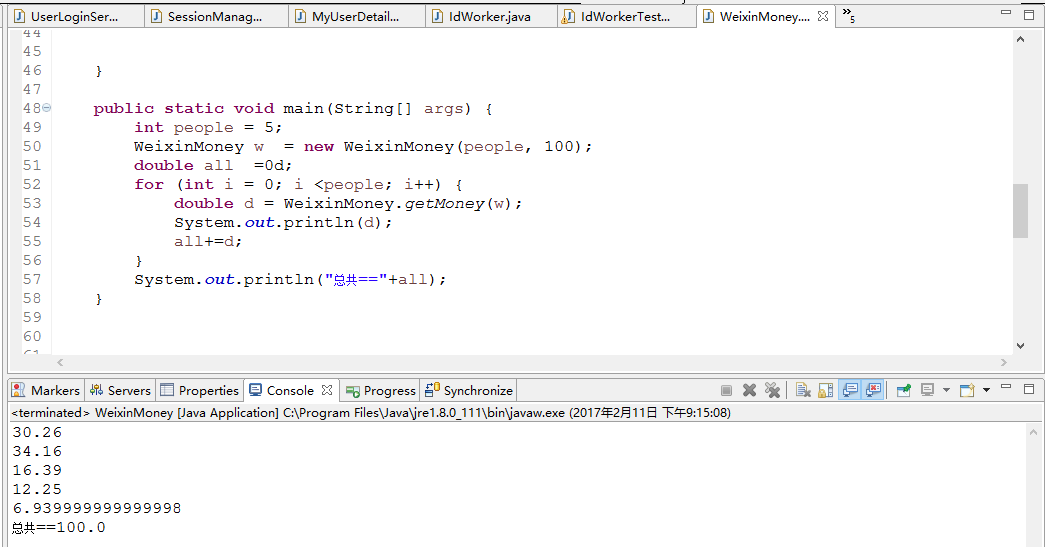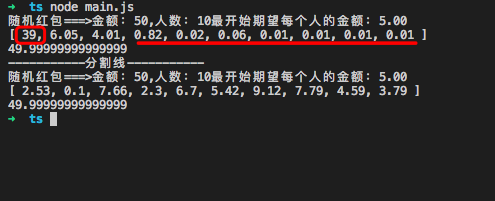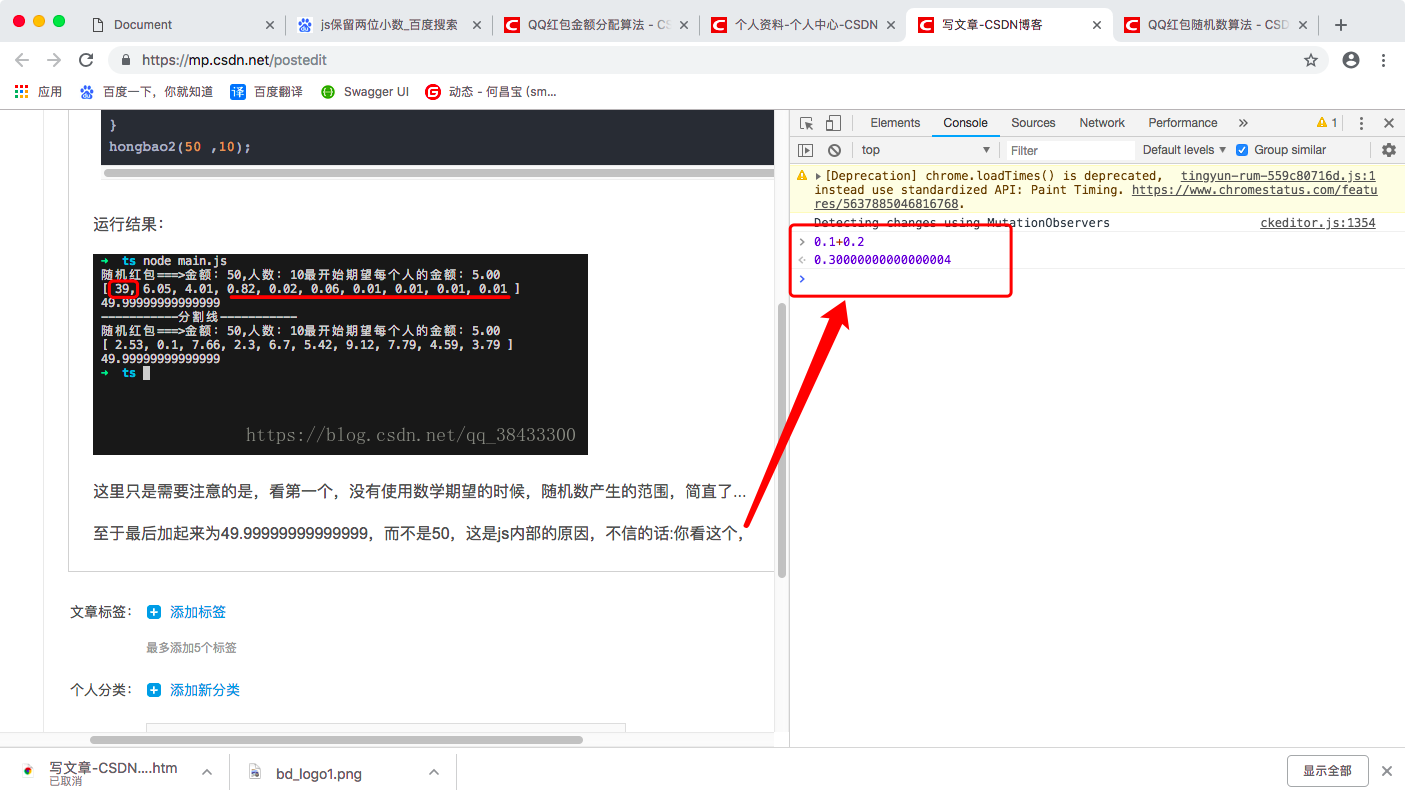• 简单实现红包随机分配,用随机的方法产生一个在 MINMONEY 到 MAXMONEY 之间的红包,并且在产生了这个红包之后需要分析数值是否合理.
•Java
• 微信红包随机分配算法初探大家平时都在使用微信收发红包，玩的不亦乐乎。别人在抢红包,而程序员在研究红包算法。那么微信红包的随机分配算法是怎么实现的呢？怎么保证没人都领得到，而且满足正态分布呢？我猜想的...
微信红包随机分配算法初探
大家平时都在使用微信收发红包，玩的不亦乐乎。别人在抢红包,而程序员在研究红包算法。那么微信红包的随机分配算法是怎么实现的呢？怎么保证没人都领得到，而且满足正态分布呢？我猜想的算法大概是这样的
红包领了不少，据观察红包主要有以下几个限制条件：
所有人都能分到红包，也就是不会出现红包数值为0的情况。所有人的红包数值加起来等于支付的金额。红包波动范围比较大，约5%~8%的红包数值在平均值的两倍以上，同时数额0.01出现的概率比较高。红包的数值是随机的，并且数值的分布近似于正态分布。

假设

比如100元，由10个人分，那么平均一个人是10元钱。然后付款后，系统开始分份儿。  第一份：系统由0～10元之间随机一个数，作为这一份的钱数，设x1。  第二份：剩下的钱(100-x1)，系统由0～(100-x1)/(10-1)随机一个数，作为这份的钱数，设x2  .。。。  第n份：剩下的钱(100-x1-x2-…-xn)，系统由0~(100-x1-x2-…-xn-1)/(10-n)随机一个数，作为这个份的钱数，设为xn。

代码块实如下
：
package com.qidi.platform.ui.logging;

import java.util.Random;
/**
*  微信红包分配算法@柒地科技
* @author 超哥
* @version v 1.0
*
*/
public class WeixinMoney {

private int peoples; //几个人分
private double allMoney; //总金额

public WeixinMoney(int  peoples,int allMoney){
this.peoples =peoples;
this.allMoney = allMoney;

}

public static double getMoney(WeixinMoney wmoney){
if (wmoney.getPeoples()==1) {
wmoney.setPeoples(0);
return wmoney.getAllMoney();
}
//随机分配算法
Random r     = new Random();
double min   = 0.01; //
double max = wmoney.getAllMoney()/wmoney.getPeoples()*2;

double money = r.nextDouble()*max;
money = money <= min ? 0.01: money;
money = Math.floor(money * 100) / 100;

wmoney.setPeoples(wmoney.getPeoples()-1);
wmoney.setAllMoney(wmoney.getAllMoney()-money);

return money;

}

public static void main(String[] args) {
int people = 5;
WeixinMoney w  = new WeixinMoney(people, 100);
double all  =0d;
for (int i = 0; i <people; i++) {
double d = WeixinMoney.getMoney(w);
System.out.println(d);
all+=d;
}
System.out.println("总共=="+all);
}

public int getPeoples() {
return peoples;
}
public void setPeoples(int peoples) {
this.peoples = peoples;
}

public double getAllMoney() {
return allMoney;
}

public void setAllMoney(double allMoney) {
this.allMoney = allMoney;
}

}展开全文算法 微信
• 闲来无事，突然想起来红包随机分配算法要怎么实现，经过一阵子思考，勉强用CAS操作和锁两种方式实现了该算法，但对于CAS实现的方式心里没底，我没办法证明算法的正确性，只能简单的重复执行该算法10000次这种恶劣的...
闲来无事，突然想起来红包随机分配算法要怎么实现，经过一阵子思考，勉强用CAS操作和锁两种方式实现了该算法，但对于CAS实现的方式心里没底，我没办法证明算法的正确性，只能简单的重复执行该算法10000次这种恶劣的方式来验证。真心希望有这方面经验的朋友可以交流一下，互相学习。还有个问题是，我把两种算法分别执行10000次的记录耗时，结果发现锁方式比CAS快，这有点出乎意料之外。但事后又想想CAS方式在竞争比较大的情况下，不停尝试然后失败确实挺耗费时间的。

package test1.Test1;

import java.math.BigDecimal;
import java.util.Iterator;
import java.util.concurrent.CountDownLatch;
import java.util.concurrent.ExecutorService;
import java.util.concurrent.Executors;
import java.util.concurrent.atomic.AtomicInteger;
import java.util.concurrent.atomic.AtomicReference;

/**
* 红包随机分配算法，每一个红包必须大于最小值小于平均值的2倍
*
*/
public class HongBaoAlgorithm {
//每个红包的最小金额
private static final double MIN = 0.01;

class HongBao{
//红包总金额
private AtomicReference<BigDecimal> amount = new AtomicReference<BigDecimal>();
//红包个数
private AtomicInteger count;
//红包总金额
private BigDecimal hbAmount;
private int hbCount;//红包个数
//上面的两对参数分别对应CAS和锁操作
public HongBao(double amount,int count){
this.amount.set(new BigDecimal(amount));
this.count = new AtomicInteger(count);
this.hbAmount = new BigDecimal(amount);
this.hbCount = count;
}

/**
* 用CAS操作随机分配一个红包
* @return
*/
public double assignHongBao(){
while(true){
//如果红包个数为0，则表示红包也被抢完，返回0
if(count.get()<=0){
return 0.0;
}
//如果是最后一个红包则直接将剩余的金额返回
if(count.get()==1){
BigDecimal c = amount.get();
if(amount.compareAndSet(c, new BigDecimal(0))){
count.decrementAndGet();
return c.setScale(2, BigDecimal.ROUND_HALF_UP).doubleValue();
}else{//如果最后一个红包金额修改失败，则表示最后一个红包已被抢走，已没有剩余红包，直接返回0
return 0.0;
}
}
BigDecimal balanceAmount = amount.get();
int balanceCount = count.get();
if(balanceCount==0){
return 0.0;
}
//求出剩余红包金额和个数的平均值
double avg = balanceAmount.divide(new BigDecimal(balanceCount),8,BigDecimal.ROUND_HALF_UP).doubleValue();
//随机获取一个MIN到2倍平均值之间的值
double cur = getRandom(MIN, avg*2);
//获取剩余金额和分配金额的差值
//由于每个红包至少是MIN这么大，此处获取剩余红包个数应该有的最小的红包金额，
//比如MIN=0.01，那么如果这次分配之后还剩2个红包，则金额至少要剩下2分钱，不然不够分
double c = new BigDecimal(balanceCount-1).multiply(new BigDecimal(MIN)).doubleValue();
//分配之后剩余金额b  需大于等于   剩余的最小值c，如果不满足则重新分配红包大小，直到满足要求
while(b < c){
cur = getRandom(MIN, avg*2);
}
//如果是最后一个红包则直接将剩余的金额返回，
//在返回结果之前再一次执行这个判断的目的是为了在多线程情况如果在返回结果之前已经被抢到只剩最后一个的时候
//还是返回随机获得金额的话则会导致总金额不会被抢完
if(count.get()==1){
BigDecimal c1 = amount.get();
if(amount.compareAndSet(c1, new BigDecimal(0))){
count.decrementAndGet();
return c1.setScale(2, BigDecimal.ROUND_HALF_UP).doubleValue();
}else{//如果最后一个红包金额修改失败，则表示最后一个红包已被抢走，已没有剩余红包，直接返回0
return 0.0;
}
}
//CAS更新金额和个数同时成功，则返回随机分配的红包金额
count.decrementAndGet();
return cur;
}

}

}

/**
* 用锁控制
* @return
*/
public synchronized double assignHongBao2(){
//如果红包个数为0，则表示红包也被抢完，返回0
if(hbCount==0){
return 0.0;
}
//如果是最后一个红包则直接将剩余的金额返回
if(hbCount==1){
hbCount--;
return hbAmount.doubleValue();
}
//求出剩余红包金额和个数的平均值
double avg = hbAmount.divide(new BigDecimal(hbCount),8,BigDecimal.ROUND_HALF_UP).doubleValue();
//随机获取一个MIN到2倍平均值之间的值
double cur = getRandom(MIN, avg*2);
//获取剩余金额和分配金额的差值
//由于每个红包至少是MIN这么大，此处获取剩余红包个数应该有的最小的红包金额，
//比如MIN=0.01，那么如果这次分配之后还剩2个红包，则金额至少要剩下2分钱，不然不够分
double c = new BigDecimal(hbCount-1).multiply(new BigDecimal(MIN)).doubleValue();
//分配之后剩余金额b  需大于等于   剩余的最小值c，如果不满足则重新分配红包大小，直到满足要求
while(b < c){
cur = getRandom(MIN, avg*2);
}
hbCount--;
return cur;
}

/**
* 计算两个数之间的随机值，结果保留两位小数
* @param begin
* @param end
* @return
*/
private double getRandom(double begin,double end){
double random = Math.random();
double amount =  random*(end - begin)+begin;
BigDecimal bg = new BigDecimal(amount);
return bg.setScale(2, BigDecimal.ROUND_HALF_UP).doubleValue();
}

}

private static void test(double hbAmount,int hbCount,int personCount) throws InterruptedException{
HongBaoAlgorithm h = new HongBaoAlgorithm();
final HongBao hb = h.new HongBao(hbAmount,hbCount);
final CountDownLatch latch = new CountDownLatch(THREAD_COUNT);
pool.execute(new Runnable() {
public void run() {
double m = hb.assignHongBao();
//					if(m>0){
//					}else{
//					}
latch.countDown();
}
});
}
pool.shutdown();
latch.await();
double amount = 0.0;
Iterator<Double> it = total.iterator();
while(it.hasNext()){
amount += it.next();
}
amount = new BigDecimal(amount).setScale(2, BigDecimal.ROUND_HALF_UP).doubleValue();
//如果分配到的总金额和传入的总金额不相等
if(amount!=hbAmount){
System.out.println("amount:"+amount);
}
//计算抢到的红包总额来判断跟设定的金额是否一致，从而判断算法的正确性，很恶劣的方式
}

public static void main(String[] args) throws InterruptedException{
long start = System.currentTimeMillis();
//执行10000次，验证一下正确率
for(int i=0;i<10000;i++){
//9个红包，10个人抢
test(100, 9, 10);
}
System.out.println("用时："+(System.currentTimeMillis()-start)+"ms");
}

}



展开全文java
• 思路： 将每个红包设置一个期望值，期望值为红包的总金额/人数，...随机出 “总人数 - 1“的红包数量， 剩下的所有金额则是最后一个红包 --------------------- 本文来自 zhaitonghui 的CSDN 博客 ，全文地址请点击...
思路：
将每个红包设置一个期望值，期望值为红包的总金额/人数， 每个红包最大金额是：期望值的两倍 。 每个红包最小金额是：0.01 。 如此循环，前面的人如果抢到红包大于期望值， 在这后面抢红包的人期望值会变小。 随机出 “总人数 - 1“的红包数量， 剩下的所有金额则是最后一个红包 --------------------- 本文来自 zhaitonghui 的CSDN 博客 ，全文地址请点击：https://blog.csdn.net/zhaitonghui/article/details/74946445?utm_source=copy
代码：
function randomMoney1(min:number,max:number):number{

const Num : number =  Math.floor((Math.random() * max) + min);
// console.log("生成范围",min,max,Num)
return Num;
}

function hongbao1(totalMoney:number,totalPerson:number){
console.log("随机红包===>金额："+totalMoney+",人数："+totalPerson+"最开始期望每个人的金额："+(totalMoney/totalPerson).toFixed(2));
let moneyArray:number[] = [];
let Money = totalMoney * 100;
let total = 0;
if(totalPerson > 1){
for(let i = 0;i<totalPerson-1;i++){
// console.log("后面还要几个人要分钱钱：",totalPerson - i - 1)
let a = randomMoney1(1,Money-(totalPerson - i - 1))
Money = (Money - a);
total += a;
moneyArray.push(a/100);
}
}
moneyArray.push((totalMoney * 100 - total)/100)
console.log(moneyArray);
//验证总数
let aaa = 0;
moneyArray.map((item)=>{
aaa+=item;
})
console.log(aaa);
}

hongbao1(50 ,10);

console.log("-----------分割线-----------");

function randomMoney2(min:number,max:number):number{
const Num : number =  Math.floor((Math.random() * max) + min);
return Num;
}
function hongbao2(totalMoney:number,totalPerson:number){
console.log("随机红包===>金额："+totalMoney+",人数："+totalPerson+"最开始期望每个人的金额："+(totalMoney/totalPerson).toFixed(2));
let moneyArray:number[] = [];
let Money = totalMoney * 100;
let total = 0;
if(totalPerson > 1){
for(let i = 0;i<totalPerson-1;i++){
//添加数学期望值
let Expect = Math.floor(Money / (totalPerson - i));
let a = randomMoney2(1,Expect*2)
Money = (Money - a);
total += a;
moneyArray.push(a/100);
}
}
moneyArray.push((totalMoney * 100 - total)/100)
console.log(moneyArray);
//验证总数
let aaa = 0;
moneyArray.map((item)=>{
aaa+=item;
})
console.log(aaa);
}
hongbao2(50 ,10);
运行结果：这里只是需要注意的是，看第一个，没有使用数学期望的时候，随机数产生的范围，简直了...
至于最后加起来为49.99999999999999，而不是50，这是js内部的原因，不信的话:你看这个，作为一个小菜鸟，我就先撤退了...大家主要看思路。
展开全文随机数
• 用度娘查了一下，看到了一个官方的回答：“随机，额度在0.01和(剩余平均值2)之间。”也就是说，每个人拿红包的时候，生成的金额都是即时计算，这样可以减少内存的占用，而每个红包的额度是在0.01和(剩余平均值2)之间...

这几天比较闲，自己研究了一下微信红包的算法。用度娘查了一下，看到了一个官方的回答：“随机，额度在0.01和(剩余平均值2)之间。”也就是说，每个人拿红包的时候，生成的金额都是即时计算，这样可以减少内存的占用，而每个红包的额度是在0.01和(剩余平均值2)之间，就是说，如果100块5个红包，第一个红包的钱会在0.01到40之间，但玩过抢红包的人都知道，这是不对的，第一个抢，拿超过剩余平均值*2是很平常的事。
而我自己在没看到这个回答之前，自己的设想是发红包后就已经将金额分配好，然后等人来打开红包。
public function index($money,$count){
if($count==1){ echo$money;exit;
}
$max=$money*100;
if($max'; } return$data;
}
我的思路是，红包总金额金额就等于一条直线的长度，然后在直线上随机放n-1个点，点与点之间的距离就等于每个红包的金额。一开始，我以为，这样会相对平均，然后我测试了100块发10个红包，测试了一万次，结果发现而且这还是我打乱点与点长度排序后所得出来的结果，如果没打乱之前的结果时这样，相差更大。
明显可以看出，离平均，差好远，是我的思路本来就是错误的吗？
回复内容：
这几天比较闲，自己研究了一下微信红包的算法。用度娘查了一下，看到了一个官方的回答：“随机，额度在0.01和(剩余平均值2)之间。”也就是说，每个人拿红包的时候，生成的金额都是即时计算，这样可以减少内存的占用，而每个红包的额度是在0.01和(剩余平均值2)之间，就是说，如果100块5个红包，第一个红包的钱会在0.01到40之间，但玩过抢红包的人都知道，这是不对的，第一个抢，拿超过剩余平均值*2是很平常的事。
而我自己在没看到这个回答之前，自己的设想是发红包后就已经将金额分配好，然后等人来打开红包。
public function index($money,$count){
if($count==1){ echo$money;exit;
}
$max=$money*100;
if($max'; } return$data;
}
我的思路是，红包总金额金额就等于一条直线的长度，然后在直线上随机放n-1个点，点与点之间的距离就等于每个红包的金额。一开始，我以为，这样会相对平均，然后我测试了100块发10个红包，测试了一万次，结果发现而且这还是我打乱点与点长度排序后所得出来的结果，如果没打乱之前的结果时这样，相差更大。
明显可以看出，离平均，差好远，是我的思路本来就是错误的吗？
看看这里的红包算法合你的要求不
https://github.com/qieangel2013/yaf
微信金额是拆的时候实时算出出来的，采用的是纯内存计算，不需要预算空间存储。采取实时计算金额的考虑：预算需要占存储，实时效率很高。

相关标签：php
本文原创发布php中文网，转载请注明出处，感谢您的尊重！

展开全文• 最近偶然间想到了红包分配问题，在这里记录下思路和代码。问题发一个N元红包，M人来分，尽量公平每个人拿到的金额。思路：假设10元红包10个人分，为了保证剩下的人能拿到红包，每个人最大能拿(红包金额-剩余人数*...
• //随机安全上限【1,limit】 取最大可用金额的平均值 limit = money - minBag*(n- i); int k = ((n-i)/2); if(n -i ) k = n -i; limit = limit/k; //limit = (money-(n-i)*minBag)/(n-i); ...
• import java.util.Random; class Scratch {  public static void main(String[] args) {  int count = 5;/个数  double amount = 200;/金额  double singleMin = 0.01;//单个红包最小金额  doub...java
• function calc(money,person){var tmp=[]for(i=0;i<person;i++){tmp.push(Math.random())}var total=eval(tmp.join('+'));var arr=[];for(i=0;i<tmp.length;i++){arr[i]=tmp[i]/total;}//console.log('ar...
• 2.(2019高二下·温州期中)条形码是按照一定编码规则排列的图形标识符。如图所示，条形码下方数字左边12位代表信息，最右边一位是校验码。校验码产生方法如下： ①把条形码下方数字从右往左依次编序号为“1,2,3,4……...
• 支持JDK1.6的随机红包分配方案，直接导入jar包即可使用。
• 1.基于Java实现的红包随机分配算法， 2.先抢后抢拿到红包的大小的期望是大致相等的
• 先假设一个红包四个人分，红包就是关于钱，那么是不是要保留两位小数，保留两位小数的方法很多，我用的方法是toFixed(2); 代码如下： function fenpei(qian){//第一个人 var one = (0.01 + Math.random()*...
• 红包不是真的随机分配哟16340020 数据科学与计算机学院目录抢红包不是真的随机分配哟 数据科学与计算机学院 对红包的独特情愫 爱爱爱不完 然而也有巨大的悲伤 当当当潜规则在这里 用户抢到的金额与用户注册时间有...数据
• <?.../* 参数请自己校验 $money 准备发送多少钱(分)$n 个数 $rate 控制红包的系数 */ function makeRedPacket($money, $n,$rate = 0.5) { //每个红包先保留1分钱 $hold =$n; //分剩下的钱...
•  (可用户发红包，点赞等逻辑)  @param allNumber  要分配的总数  @param toPart    要分配的份数  */ - ( void )randomNumber:( NSUInteger )allNumber toPart:( ...iOS
• 可以看出，红包随机金额生成的输入是一个四元组 <sum, num, min, max>，其中 sum 是红包总额，num 是红包数量，min 和 max 分别是红包最小最大值。所以这里可以抽象成如下算法： input: <sum, num, min, ...
• * 随机红包 * @param number total 红包金额 * @param int num 拆成的个数 * @return array */ function open_red(total,total,total, num) { min=0.01;//每个人最少能收到0.01元min=0.01;//每个人最少...
• 你可能遇到过这样的情况，老板把你和小张拖到一个群发红包慰劳一下，这个微信红包价值200元，你眼疾手快，先点开，一看：摔！0.01元！小张乐呵呵地领了剩下的红包。 虽然200元丢在路上你都不一定心动（只是假设下，...
• ## Java 随机红包、平均红包分配规则

千次阅读 热门讨论 2018-10-19 16:16:53
import java.util.ArrayList; import java.util.List;... * 红包分配规则 * * @author houzh * */ public class RedPacketUtils { // 最小红包额度 private static final int MINMONEY = 1;...
• 方法1 <?... $total = 7;//红包总金额，单位：分（避免...// 分成6个红包，支持6人随机领取$moneyt=0.00; for ($i = 0;$i < $number;$i++) { $max =$total / ($number -$i) * 2 - 1; if (\$max == 1){ ...PHP
• 输入红包金额\数量及红包最小值,产生随机分配方案. 这是控制随即空间的一种方法.
• 主要为大家详细介绍了php版的微信红包随机生成算法，具有一定的参考价值，感兴趣的小伙伴们可以参考一下
• 过年会有个小游戏，6个人每次发红包6元分成6份 ，拿最大红包的继续发。 首先是最简单的想法 ，在范围内6元减去0.01元*5取个随机数 ，然后按照每人每次六分之一概率抽到最大红包。这是从第一视角 单纯考虑每次得失来...
• 在做一个项目的随机红包金额时，需要随机分配一个奖池的金额，查了其他人写的随机算法，要么分配极不平均，要么不能全部分完奖池，于是自己写了一个 核心思想是每次都按剩余的平均数随机，得到结果比较理想，记录......